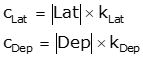### a. Compass RuleEquations K-3 and K-4Equations K-5 and -6

### b.  Transit RuleEquations K-7 and K-8Equations K-9 and K-10Equation K-11Equation K-12Equations K-13 and K-14